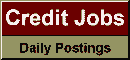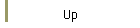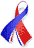DefaultRisk.com the web's biggest credit risk modeling resource.Export citation to:- HTML- Text (plain)- BibTeX- RIS- ReDIF

Quantile Mechanics

by György Steinbrecher of the University of Craiova, Romania, and
William T. Shaw of King's College London

July 16, 2007

Abstract: In both modern stochastic analysis and more traditional probability and statistics, one way of characterizing a static or dynamic distribution is through its quantile function. A direct understanding of this function offers tangible benefits not available directly from the density function. For example, the simplest way of simulating any non-uniform random variable is by applying its quantile function to uniform deviates. Modern methods of Monte-Carlo simulation, techniques based on low-discrepancy sequences and copula methods all call for the use of quantile functions of marginal distributions. We propose a new approach to quantiles based first, for the static case, on non-linear ordinary differential equations, which we solve by the classical method of power series with easily computed non-linear recursion relations for the coefficients. These methods provide accuracy beyond standard machine precision on subsets of the unit interval and are well adapted to the benchmarking of approximate techniques, and, for some cases, live simulation. We provide some web links to implementations that may be starting points for the inclusion of these algorithms in simulation methods. Next, we consider the time evolution of quantiles via the quantilized Fokker-Planck equation. This is a non-linear partial differential equation and we are able to utilize the static formulation to provide elementary time-dependent and equilibrium solutions.

Keywords: Inverse CDF, quantile function, normal, Student, beta, T-distribution, simulation, Monte Carlo, inverse cumulative distribution function, non linear ordinary differential equations, recurrence relations, Fokker-Planck equation, stochastic differential equation, partial differential equation.

Published in: European Journal of Applied Mathematics, Vol. 19, No. 2, (April 2008), pp. 87-112.

Previously titled: Differential Equations for Quantile Functions

Books Referenced in this paper:  (what is this?)